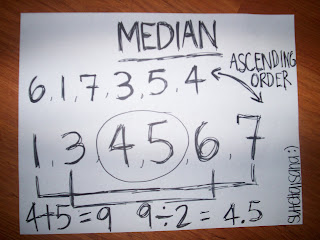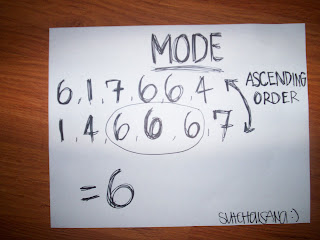### Sutchai's Measures of Central Tendency

Monday, September 29, 2008
Mean:In a data set, the sum of all the data points, divided by the number of data points; average.Median:The middle number in a data set when the data are put in order; a type of average.Mode:A type of average; the number (or numbers) that occurs most frequently in a set of data.Range:In statistics, the difference between the largest and the smallest numbers in a data set.Here's a video that explains the mean, median, mode, and range :